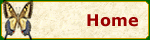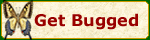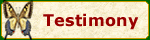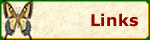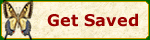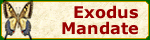Articles

Ants and the Golden Ratio

By Karl Priest December 14, 2012 (revised 3-6-15)

The Golden Ratio (alsocalled the Golden Section, Medial Section, Golden Mean, Divine Section, and Divine Proportion) is when the ratio of the sum of the quantities to the larger quantity is equal to the ratio of the larger quantity to the smaller one .

Euclid (ca 300 B.C.) studied it. (He called it dividing the line in mean and extreme ratio).

The ratio has been used hundreds of years by artists and architects for beauty and balance in design.

Take a line (A) and divide it into two parts, B (long part) and C (short part). The ratio of short part to long part should be the same as the ratio of long part to the whole line. In other words, as the short part is to the long part, so is the long part to the whole line. (C is to B as B is to A)

C:B = B:A or C/B = B/A is same as B:A = C:B or B/A = C/B

B = 0.618 C = 0.382

From this can be solved that B = (√5 + 1)/2 × C or B ≈ 1.618 × C. This number (√5 + 1)/2 is signified by the Greek letter Phi (φ ). If you divide the line so that longer part is Phi times (1.618033988749895… or about 1.62) the shorter part, you've divided it in the golden section (or golden cut). Note: √5 ≈ 2.236

Divide the line again and again in the same way and combine the segments to create a measuring stick:

The body sections (and leg sections) of an ant are defined by the golden sections of its length. Here are diagrams showing the body sections.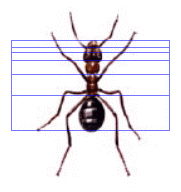The Golden Ratio is also found in the Fibonacci Sequence, the human body, the Mona Lisa, the Great Pyramid, the Parthenon, plants, Davinci’s Vetruvian Man, shells, DNA, and galaxies and more! It is awesome! Search the Internet for Golden Ratio images or start at “Mathematical Thought” http://math2033.uark.edu/wiki/index.php/Golden_ratio.

Compiled from:

http://www.homeschoolmath.net/teaching/fibonacci_golden_section.php

http://www.goldennumber.net/golden-section/

http://www.goldennumber.net/golden-ratio/

http://en.wikipedia.org/wiki/Golden_ratio

http://www.goldennumber.net/nature/

http://phi-number.blogfa.com/

http://www.goldennumber.net/what-is-phi/

1. Some insects display a rudimentary sense of numbers, such as the solitary wasps that prey upon a single species. The mother wasp lays her eggs in individual cells and provides each egg with a number of live caterpillars on which the young feed when hatched. Some species of wasp always provide five, others twelve, and others as high as twenty-four caterpillars per cell. The number of caterpillars is different among species, but always the same for each sex of larva. The male solitary wasp in the genus Eumenes is smaller than the female, so the mother of one species supplies him with only five caterpillars; the larger female receives ten caterpillars in her cell.
http://en.wikipedia.org/wiki/Insect#Sound_production_and_hearing

2. Phi is Fantastic.

Phi’s “cousin” pi is fun too, and used by skeptics to scoff at the Bible. Does the Bible Contain a Mathematically Incorrect Value for "Pi"? Here is another slice of lemon pi pie for skeptics.

3. Another buggy math article: Do Cicadas Count?

4. Some scientists say we live in a "Golden Ratio" universe and 1.618 is “ the key to unifying all of science.”

5. “(I)t was discovered that the Golden Ratio did relate to butterfly feeding habits, and sap-feeding butterflies have proboscis conformations that closely resemble the Golden Ratio.”

---------------------

The math lesson that destroys evolutionism: Middle School Lesson Plan